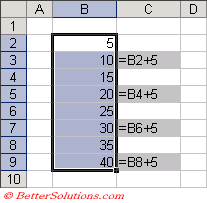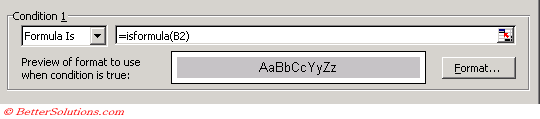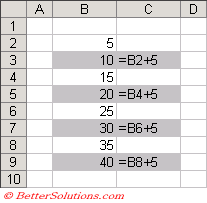# VBA Function

This example shows you how to highlight all the cells which contain a formula.
It is not possible to identify which cells containing formulas using the regular worksheet functions so we need to create our own custom function.
To create this custom worksheet function you need to insert a code module into the VBA Project and insert the following code.

`Public Function IsFormula(rgeRange As Range) As Boolean    IsFormula = rgeRange.HasFormula End Function `

This function returns the value True if the cell reference passed to the function contains a formula and False otherwise.

### Highlight the cells

Select the cells you want to apply the conditional formatting to, in this case "B2:B9".### Enter the Conditions

Press (Format > Conditional Formatting) to display the Conditional Formatting dialog box.
Select the "Formula is" in the first drop-down list and enter the formula "=isformula(B2)".
Sometimes when you add a custom function in the Conditional Formatting dialog box Excel will put speech marks around your formula.
These must be removed if they appear.Press OK to apply the conditional formatting.